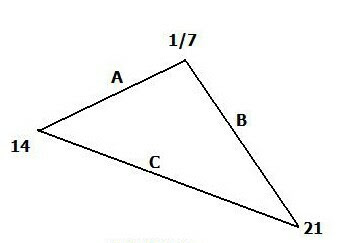## Saturday, January 23, 2010We now return to the p-adics, the novel breed of numbers introduced in the Jan. 13 entry. We left off with three problems related to the content of that article. To refresh, the 3 problems were:

1-- For the triangle in Fig. 2, use 7-adic absolute values applied to the sides of the triangle, thereby compute: A, B and C and show it is isosceles.

2- Find the value of the sum S for: S = 1 + 7 + (7)^2 + (7)^3 + (7)^4 + (7)^5 + .......

3- Using (2) as written, "invent" a new irrational number based on the p-adic form.
---

For convenience, I've reproduced Fig. 2 from the original blog piece, which we can work with in the course of the solution.

1-

Find the 7-adic value for each side;

[A]_7 = [14 - 1/7]_7 = abs{[2 x 7]_7 - 1/[1 x 7]_7}
= abs[1/7 - 7] = 6 6/7 = 48/7

[B]_7 = [1/7 - 21]_7 = abs{[1/[1 x 7]_7 - [3 x 7]_7}
= abs[7 - 1/7] = 6 6/7 = 48/7

[C]_7 = [21 - 14]_7 = abs{[3 x 7] _7 - [2 x 7]_7} = abs{[1/7 - 1/7]_7} = _7 = 1

and from these calculations of p-adic (7-adic) absolute values we find side A = side B = 48/7, hence in the p-adic format the triangle is isosceles.

2- S = 1 + 7 + (7)^2 + (7)^3 + (7)^4 + (7)^5 + .......

So rewrite the sum (multiplying both sides by 7):

7S = 7 + (7)^2 + (7)^3 + (7)^4 + (7)^5 + (7)^6 + .........

Subtract the lower form from the upper, viz.:

S = 1 + 7 + (7)^2 + (7)^3 + (7)^4 + (7)^5 + .......

-7S = 7 + (7)^2 + (7)^3 + (7)^4 + (7)^5 + (7)^6 + .........
________________________________________

S - 7S = 1 (all other top and bottom terms cancel)

whence:
-6S = 1 and therefore, S = -1/6

Problem 3- We want to use the prior number to invent a new irrational number. The most direct way is to use the same (7-adic) form, and simply increase powers in a different way:

Thus: let N - the new irrational be S' where S' is a variant of S such that:

N=7 + (7)^3+ (7)^6 + (7)^9 + (7)^12 + (7)^15 + .........